Views:

Description
This article describes how numerical engines interpret a tricky 1D-2D model configuration where the 1D model network outlet is set to a level under the 2D Terrain level.

The process is as described below.

#01 - In a 1D-2D Storm Water Model there is a 1D outlet level below the 2D ground level - see Fig. 1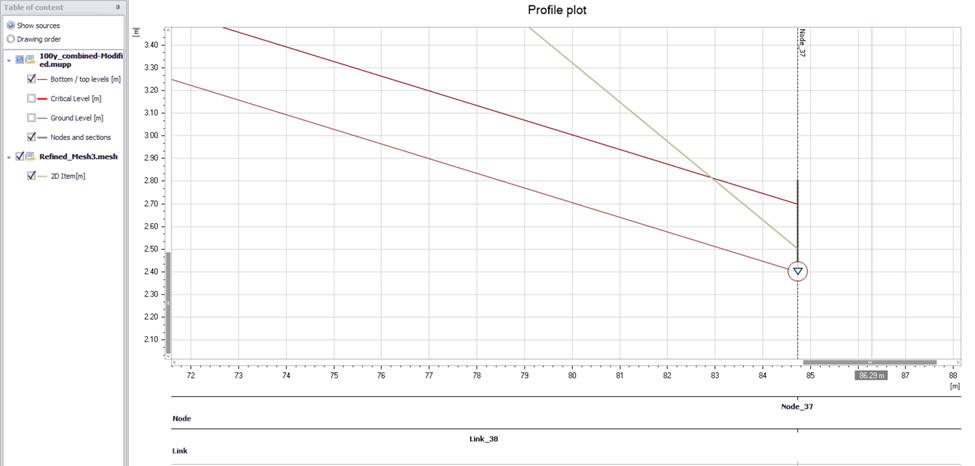Fig. 1 - Example of a configuration where the outfall (Node 37) falls under the 2D terrain model (green line)

#02 - A warning gives an idea of the potential issues that this configuration may represent- see Fig. 2

One would expect the model to fail and launch an error under these circumstances, but instead the model runs flagging one single warning at this location. The warning message indicates that there is a risk of instabilities and could lead to mass errors in the water balance.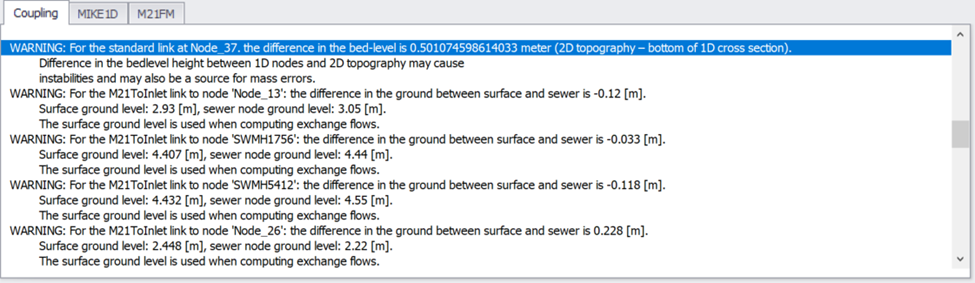Fig. 2 – Warnings emitted during simulation launch

#03 - When viewing results at the outlet, it is noticed that the water level has not reached ground level and yet there is a positive flow to the surface out via the coupled outlet (Node_37 in the images below and note the peak water level vs 2D ground level) - see Fig. 3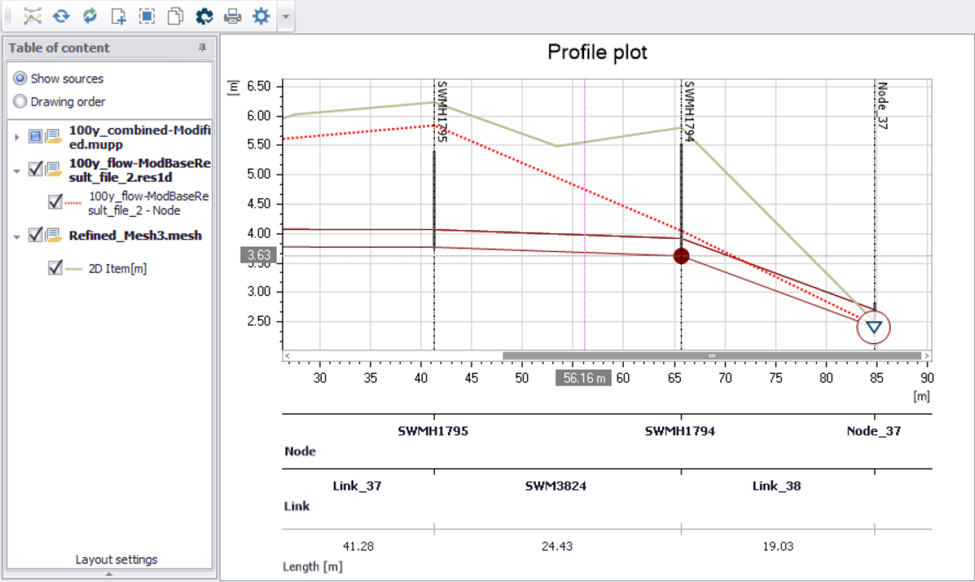Fig. 3 - The water level results showing the levels under the 2D terrain

#04 - Under these hydraulic conditions the discharge to the surface is unexpected, however, if one looks at the Discharge to Surface results at this location it is found that this discharge is positive - see Fig. 4 - Fig. 5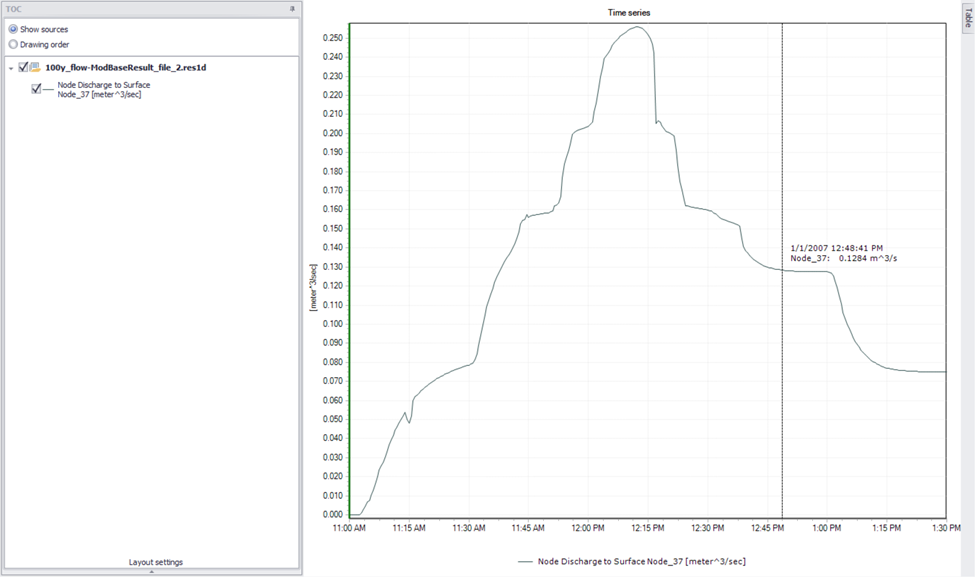Fig. 4 - The outlet node shows positive discharge to the 2D surface

As a result, there are some issues with the modelers' comprehension of the scenario.

• What are the hydraulic calculations doing here, where flow can escape through the outlet despite the computed water level being lower than ground level?
• Why does the model accept a 1D pipe outlet placed below the 1D ground level without throwing an error?

Note: To understand this condition, it is important to note that

A. The 2D water depth is not used - or rather, it is used as a boundary condition in the 1D outlet, only when the 2D level is higher than the 1D bottom level.

B. The water depth on 2D surface is lower than 0.1 [m]

When these two conditions (A+B) are fulfilled (simultaneously), the water depth in 1D outlet is not used straight but linearized between 0.0 and the fraction of (0.1 + delta bottoms), in order to make a smooth transition to a dry or partially filled 1D link.

In order to make a smooth transition to a dry or partially filled 1D link when these two circumstances are met (concurrently), the water depth in the 1D outflow is linearized between 0.0 and the fraction of (0.1 + delta bottoms) instead of being utilized straight.

Thus, if the water depth on 2D surface is 5 centimeters (half of the limit 0f 0.1 meters), and the bottom level difference is 0.5 meters (i.e. 1D outlet is 0.5 meter below the 2D surface), the water level applied would be equivalent of a water depth, calculated as:

0.05/0.1*(0.5+0.1) = 0.3 [m] + 1D outlet invert level.

This is 0.2 meters below the 2D ground level.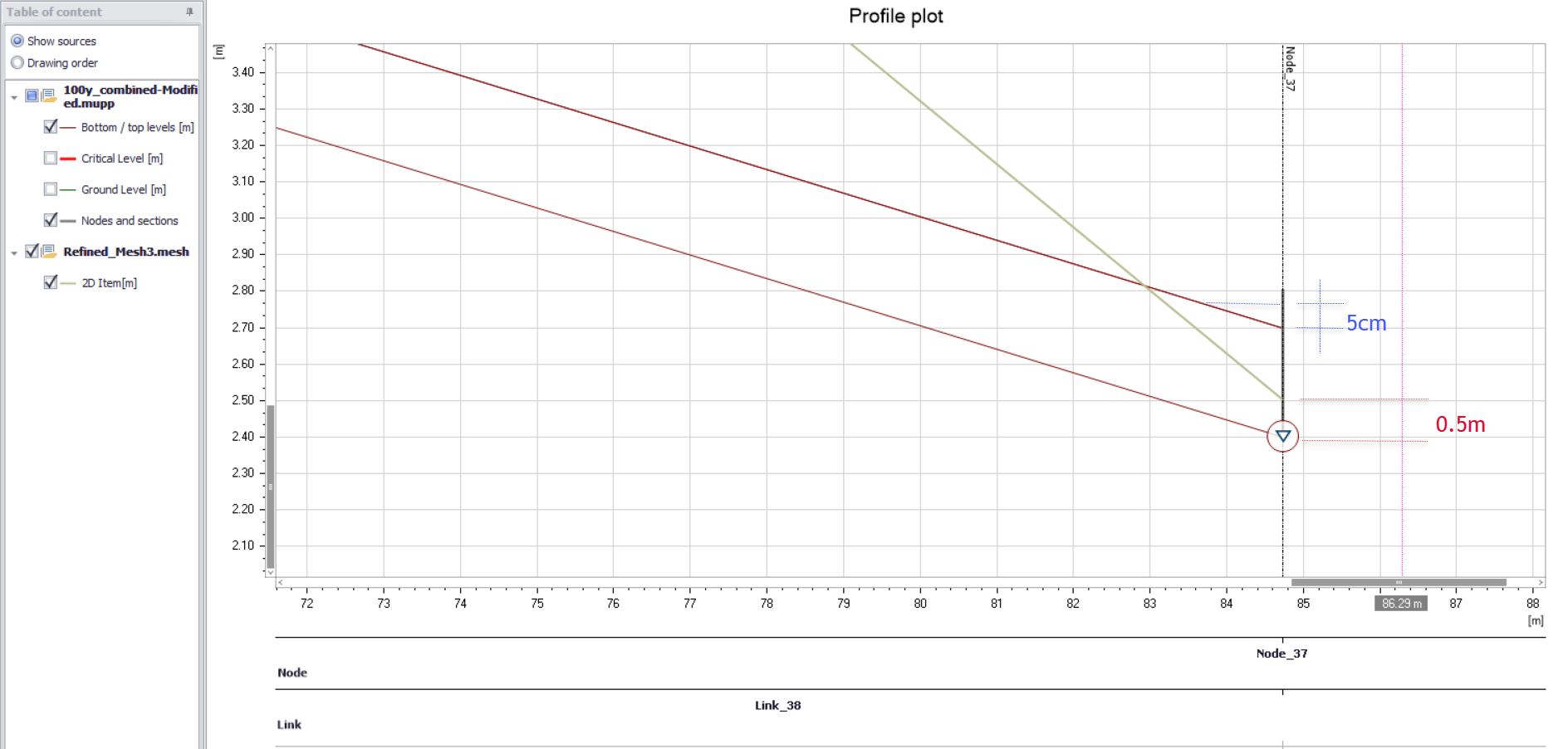Fig. 5 - Water level on 2D at 5 cm with a difference of 0.5 meters between invert level and 2D ground level

If the 2D water depth was 0.01[m], the water level applied would be equivalent to a water depth of 0.06 m [m], i.e., 46 cm below 2D surface. This "treatment" is applied to secure a stable simulation and correct the mass balance. In all other cases, the straight 2D water level is used (down to the 1D bottom level).

Conclusion
This type of situation calls for proper attention when setting up a model and running simulations. The warning issued by the program clearly indicates that the setup is not correct. In such cases, it is recommended either to lift the 1D outlet to the 2D ground surface level or to modify the Digital Elevation Model so that in actually includes a local "hole" where the outlet is located.